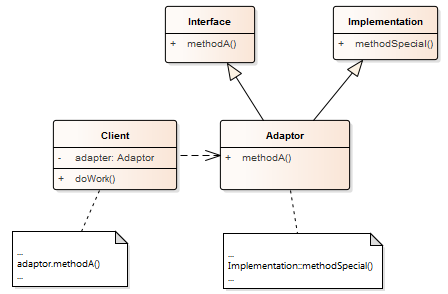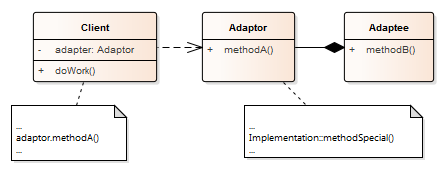The idea of the adapter pattern is straightforward: It converts the interface of a class into another interface.Imagine you have a class that implements the functionality required by the client. There is only one issue: the interface does not follow your company’s naming policy. Thanks to the Adapter Pattern, you can pretty easily support the required interface using the existing class.

The Adapter Pattern is the only pattern inside the book “Design Patterns: Elements of Reusable Object-Oriented Software”  (Design Pattern) that is implemented on a class level but also on an object level. Here are the facts before I show you both ways to implement this structural pattern.

### Purpose

• Translate one interface into another one

• Wrapper

### Use Case

• A class does not have the required interface
• Definition of a general interface for a set of similar classes

### Example

The container adapters s`td::stack``, std::queue,` and` std::priority_queue` provide a different interface for the sequence containers. The following code snippet shows the template signature of the three container adapters:

```template<typename T, typename Container = std::deque<T>>
class stack;

template<typename T, typename Container = std::deque<T>>
class queue;

template<typename T, typename Container = std::vector<T>,
typename Compare = std::less<typename Container::value_type>>
class priority_queue;
```

By default,` std::stack,` and `std::queue` use `std::deque` as sequence container, but `std::priority_queue` uses` std::vector`. Additionally, `std::priority_queue `also requires a comparator that is defaulted to `std::less` of the sequence container elements.

##Modernes C++ Mentoring

Be part of my mentoring programs:

• "Fundamentals for C++ Professionals" (open)
• "Design Patterns and Architectural Patterns with C++" (open)
• "C++20: Get the Details" (reopens December 2023)
• Do you want to stay informed about my mentoring programs: Subscribe via E-Mail.

C++ supports insert iterators and streams iterators.

• Insert Iterators

With the three insert iterators` std::front_inserter`, `std::back_inserter`, and `std::inserter,` you can insert an element into a container at the beginning, at the end or an arbitrary position, respectively.

• Stream Iterators

Stream iterator adapters can use streams as a data source or data sink. C++ offers two functions to create istream iterators and two to create ostream iterators. The created istream iterators behave like input iterators, the ostream iterators like insert iterators.

### Structure

The following two class diagrams show the structure of the Adapter Pattern, based on classes or on objects. For short, I call them class adapters and object adapters.`Client`

• Uses the member function `methodA()` of the `Adapter`

`Adaptor`

• Class
• Provides the functionality of `methodA()` using multiple inheritances
• Is publicly derived from `Interface` and privately from `Implementation`
• Object
• Delegates the member function call to its member `Adaptee`

`Adaptee`

• Implements the functionality of the client

Now, the class or object based Adapter Pattern should be straightforward.

## Implementation

In the following example, the class `RectangleAdapter` adapts the interface of the` LegacyRectangle`.

```// adapterClass.cpp

#include <iostream>

typedef int Coordinate;
typedef int Dimension;

class Rectangle {
public:
virtual void draw() = 0;
virtual ~Rectangle() = default;
};

class LegacyRectangle {
public:
LegacyRectangle(Coordinate x1, Coordinate y1, Coordinate x2, Coordinate y2) : x1_(x1), y1_(y1), x2_(x2), y2_(y2){
std::cout << "LegacyRectangle:  create.  (" << x1_ << "," << y1_ << ") => ("
<< x2_ << "," << y2_ << ")" << '\n';
}

void oldDraw() {
std::cout << "LegacyRectangle:  oldDraw.  (" << x1_ << "," << y1_
<< ") => (" << x2_ << "," << y2_ << ")" << '\n';
}

private:
Coordinate x1_;
Coordinate y1_;
Coordinate x2_;
Coordinate y2_;
};

class RectangleAdapter : public Rectangle, private LegacyRectangle {
public:
RectangleAdapter(Coordinate x, Coordinate y, Dimension w, Dimension h) : LegacyRectangle(x, y, x + w, y + h) {  // (1)
std::cout << "RectangleAdapter: create.  (" << x << "," << y
<< "), width = " << w << ", height = " << h << '\n';
}

void draw() override {
oldDraw();
std::cout << "RectangleAdapter: draw." << '\n';
}
};

int main() {

std::cout << '\n';

Rectangle* r = new RectangleAdapter(120, 200, 60, 40);
r->draw();

delete r;

std::cout << '\n';

}
```

`RectangleAdapter` derived the interface from the `Rectangle`, and the implementation for `LegacyRectangle `using multiple inheritances. Additionally, `RectangleAdapter` adapts the size of the `LegacyRectangle` (line 1).

This implementation of the Adapter Pattern is one of the rare use cases for private inheritance. Let me write a few words about interface inheritance and implementation inheritance.

• Interface inheritance uses public inheritance. It separates users from implementations to allow derived classes to be added and changed without affecting the users of the base class. Derived classes support the interface of the base class.
• Implementation inheritance often uses private inheritance. Typically, the derived class provides its functionality by adapting functionality from the base class. Derived classes don’t support the interface of the base class.

Finally, here is the output of the program:In the following implementation, the `RectangleAdapter` delegates its calls to its adaptee `LegacyRectangle`.

```// adapterObject.cpp

#include <iostream>

typedef int Coordinate;
typedef int Dimension;

class LegacyRectangle {
public:
LegacyRectangle(Coordinate x1, Coordinate y1, Coordinate x2, Coordinate y2) : x1_(x1), y1_(y1), x2_(x2), y2_(y2){
std::cout << "LegacyRectangle:  create.  (" << x1_ << "," << y1_ << ") => ("
<< x2_ << "," << y2_ << ")" << '\n';
}

void oldDraw() {
std::cout << "LegacyRectangle:  oldDraw.  (" << x1_ << "," << y1_
<< ") => (" << x2_ << "," << y2_ << ")" << '\n';
}

private:
Coordinate x1_;
Coordinate y1_;
Coordinate x2_;
Coordinate y2_;
};

public:
RectangleAdapter(Coordinate x, Coordinate y, Dimension w, Dimension h) : legacyRectangle{LegacyRectangle(x, y, x + w, y + h)} {  // (1)
std::cout << "RectangleAdapter: create.  (" << x << "," << y
<< "), width = " << w << ", height = " << h << '\n';
}

void draw() {
legacyRectangle.oldDraw();
std::cout << "RectangleAdapter: draw." << '\n';
}
private:
LegacyRectangle legacyRectangle;
};

int main() {

std::cout << '\n';

r.draw();

std::cout << '\n';
}
```

The class `RectangleAdapter` creates it `LegacyRectangle` directly in its constructor (line 1). Another option would be to make `LegacyRectangle ` a constructor parameter of` RectangleAdapter:`

```class RectangleAdapter {
public:
...
};

```

The output of this program is identical to the previous one.

### Related Patterns

• The Bridge Pattern is similar to the object adapter but has a different intent. The Bridge Pattern’s purpose is to separate the interface from the implementation, but the adapter’s purpose is to modify an existing interface.
• The Decorator Pattern extends an object without changing its interface. Decorators are pluggable but not bridges or adapters.
• The Proxy Pattern extends the implementation for the object it stands for but doesn’t change its interface.

The class adapter applies classes and their subclasses. It uses the separation of interface and implementation and runtime dispatch with virtual function calls. Its functionality is hard-coded and available at compile time. The class adapter provides less flexibility and dynamic behavior, such as the object adapter.

The object adapter uses the relationship of objects.

You build your abstraction by composing objects and delegating their work. This composition can be done at runtime. Consequentially, an object adapter is more flexible and allows it to exchange the delegated object at run time.

## What’s Next?

The Bridge Pattern helps to separate the interface from its implementation. Let me introduce it in my next post.

Thanks a lot to my Patreon Supporters: Matt Braun, Roman Postanciuc, Tobias Zindl, G Prvulovic, Reinhold Dröge, Abernitzke, Frank Grimm, Sakib, Broeserl, António Pina, Sergey Agafyin, Андрей Бурмистров, Jake, GS, Lawton Shoemake, Jozo Leko, John Breland, Venkat Nandam, Jose Francisco, Douglas Tinkham, Kuchlong Kuchlong, Robert Blanch, Truels Wissneth, Kris Kafka, Mario Luoni, Friedrich Huber, lennonli, Pramod Tikare Muralidhara, Peter Ware, Daniel Hufschläger, Alessandro Pezzato, Bob Perry, Satish Vangipuram, Andi Ireland, Richard Ohnemus, Michael Dunsky, Leo Goodstadt, John Wiederhirn, Yacob Cohen-Arazi, Florian Tischler, Robin Furness, Michael Young, Holger Detering, Bernd Mühlhaus, Matthieu Bolt, Stephen Kelley, Kyle Dean, Tusar Palauri, Dmitry Farberov, Juan Dent, George Liao, Daniel Ceperley, Jon T Hess, Stephen Totten, Wolfgang Fütterer, Matthias Grün, Phillip Diekmann, Ben Atakora, Ann Shatoff, Rob North, Bhavith C Achar, and Marco Parri Empoli.

Thanks, in particular, to Jon Hess, Lakshman, Christian Wittenhorst, Sherhy Pyton, Dendi Suhubdy, Sudhakar Belagurusamy, Richard Sargeant, Rusty Fleming, John Nebel, Mipko, Alicja Kaminska, Slavko Radman, and David Poole.

 My special thanks to EmbarcaderoMy special thanks to PVS-StudioMy special thanks to Tipi.buildMy special thanks to Take Up Code## Seminars

I’m happy to give online seminars or face-to-face seminars worldwide. Please call me if you have any questions.

### Standard Seminars (English/German)

Here is a compilation of my standard seminars. These seminars are only meant to give you a first orientation.

• C++ – The Core Language
• C++ – The Standard Library
• C++ – Compact
• C++11 and C++14
• Concurrency with Modern C++
• Design Pattern and Architectural Pattern with C++
• Embedded Programming with Modern C++
• Generic Programming (Templates) with C++

#### New

• Clean Code with Modern C++
• C++20

### Contact Me

Modernes C++ Mentoring,Tags:
0 replies